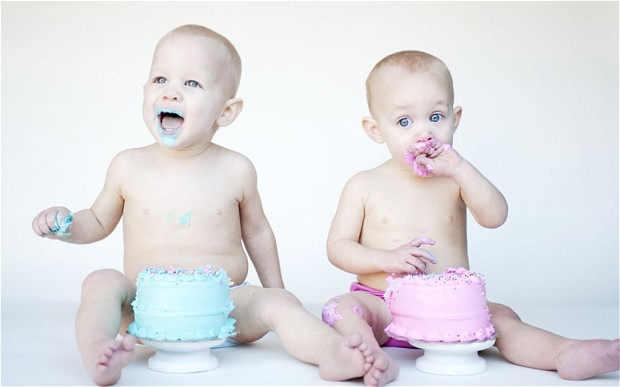# Chinese Gender Chart: Predict Your Baby’s Sex

1649As legend would have it, the Chinese Gender Chart was buried in a royal tomb over 700 years ago. Recently discovered, the chart has been used to predict the gender of an unborn child based on the mother’s age and month of conception.

Simply find the age of the mother at the time of conception on the chart below and then follow across to the month the baby was conceived to find the predicted gender. Although this chart is said to have a success rate of over 90 percent, nothing is perfect. But check out your own kids and see if its accurate, then suggest to would be mothers!

 Jan Feb Mar Apr May Jun Jul Aug Sept Oct Nov Dec 18 G B G B B B B B B B B B 19 B G B G G B B G B B G G 20 G B G B B B B B B G B B 21 B G G G G G G G G G G G 22 G B B G B G G B G G G G 23 B B B G B B G G G B B G 24 B G G B B G B G B B G B 25 G B G B G B G B G B B B 26 B B B B B G B G G B G G 27 G G B B G B G G B G B B 28 B B B G G B G B G G B G 29 G B G G B G G B G B G G 30 B B G B G B B B B B B B 31 B B B B G G B G B G G G 32 B G G B G B B G B B G B 33 G B B G G B G B G B B G 34 B B G G B G B B G B G G 35 B G B G B G B G B B G B 36 B G B B B G B B G G G G 37 G G B G G G B G G B B B 38 B B G G B G G B G G B G 39 G G B G G G B G B B G B 40 B B B G B G B G B G G B 41 G G B G B B G G B G B G 42 B G G B B B B B G B G B 43 G B G G B B B G G G B B 44 B G G G B G B B G B G B 45 G B G B G G B G B G B G